# RRB ALP 2018 Practice Test Papers | Arithmetic Questions (Day-29)

Dear Aspirants, Here we have given the Important RRB ALP & Technicians Exam 2018 Practice Test Papers. Candidates those who are preparing for RRB ALP 2018 can practice these Arithmetic Questions to get more confidence to Crack RRB 2018 Examination.

[WpProQuiz 1963]

Click “Start Quiz” to attend these Questions and view Explanation

1. If the sum of the length, breadth and height of a rectangular parallelopiped is 24 cm and the length of its diagonal is 15 cm, then its total surface area is

(a) 351 cm2

(b) 256 cm2

(c) 265 cm2

(d) 315 cm2

1. Two successive discounts of 70% and 30% are equivalent to a single discount of

(a) 89%

(b) 75%

(c) 79%

(d) 100%

1. A merchant allows a discount of 10% on marked price for the cash payment. To make a profit of 17%, he must mark his goods higher than their cost price by

(a) 30%

(b) 33%

(c) 40%

(d) 27%

1. A person takes a loan of Rs.10,000 partly from a bank at 8% p.a. and remaining from another bank at 10% p.a. He pays a total interest of Rs.950 per annum. Amount of loan taken from the first bank (in Rs.) is

(a) 2500

(b) 5200

(c) 2050

(d) 5020

1. The sum of the present ages of father and son is 90 years. 10 years earlier the ratio of their ages was 5: 2. The present age of the father is:

(a) 65

(b) 68

(c) 70

(d) 60

1. A,B and C contract a work for Rs. 440. Together A and B are to do 9/11 of the work. The share of C should be:

(a) 75

(b) 90

(c) 100

(d) 80

1. A number when reduced by 10% gives 30. The number is

(a) 35

(b) 331/2

(c) 331/3

(d) 40

1. The present ages of two persons are 36 and 50 years respectively, if after n years the ratio of their ages will be 3: 4, then the value of n is

(a) 3

(b) 4

(c) 7

(d) 6

1. Out of 20 boys, 6 are each of 1 m 15 cm height, 8 are of 1 m 10 cm and rest of 1 m 12 cm. The average height of all of them is

(a) 1 m 12 cm

(b) 1 m 12.1 cm

(c) 1 m 21.1 cm

(d) 1 m 21 cm

1.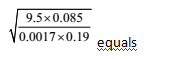(a) 5

(b) 50

(c) 500

(d) 0.05

1. Answer: (a)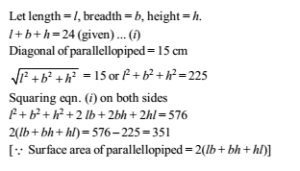30/100×70/100=21/100 Which implies 79% discount.

Solving this type of question by short cut.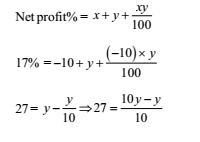27 × 10 = 9y

y = 30%

Hence, He must mark his goods 30% higher than their cost price.

1. Answer: (a)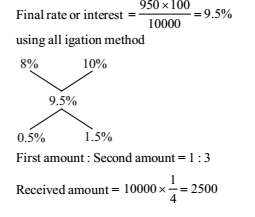Let present age of father = x

present age of son = y

According to question, x + y = 90 …(i)

Now, (x -10 )/( y- 10)=5/ 2

2x – 20 = 5y – 50

2x – 5y = –30 …(ii)

from eq. (i) and (ii) x = 60 y = 30

The present age of mother = 60 years.

Remaining work = 1– 9/11 =2/11

C will get = 2/11 ×440 = 2× 40 = 80

Number=30/90×100=33 1/3

According to question

36+n/( 50+n)  = ¾

36 × 4 + 4n = 50 × 3 + 3n

4n – 3n = 150 – 144

n = 6

1. Answer: (b)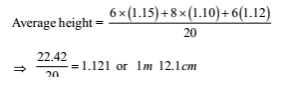2. Answer: (b)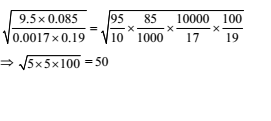RRB ALP 2018 Practice Test Papers | Arithmetic Questions (Day-1)

RRB ALP 2018 Practice Test Papers | Arithmetic Questions (Day-2)

RRB ALP 2018 Practice Test Papers | Arithmetic Questions (Day-3)

RRB ALP 2018 Practice Test Papers | Arithmetic Questions (Day-4)

RRB ALP 2018 Practice Test Papers | Arithmetic Questions (Day-5)

RRB ALP 2018 Practice Test Papers | Arithmetic Questions (Day-6)

RRB ALP 2018 Practice Test Papers | Arithmetic Questions (Day-7)

RRB ALP 2018 Practice Test Papers | Arithmetic Questions (Day-8)

RRB ALP 2018 Practice Test Papers | Arithmetic Questions (Day-9)

RRB ALP 2018 Practice Test Papers | Arithmetic Questions (Day-10)

RRB ALP 2018 Practice Test Papers | Arithmetic Questions (Day-11)

RRB ALP 2018 Practice Test Papers | Arithmetic Questions (Day-12)

RRB ALP 2018 Practice Test Papers | Arithmetic Questions (Day-13)

RRB ALP 2018 Practice Test Papers | Arithmetic Questions (Day-14)

RRB ALP 2018 Practice Test Papers | Arithmetic Questions (Day-15)

RRB ALP 2018 Practice Test Papers | Arithmetic Questions (Day-16)

RRB ALP 2018 Practice Test Papers | Arithmetic Questions (Day-17)

RRB ALP 2018 Practice Test Papers | Arithmetic Questions (Day-18)

RRB ALP 2018 Practice Test Papers | Arithmetic Questions (Day-19)

RRB ALP 2018 Practice Test Papers | Arithmetic Questions (Day-20)

RRB ALP 2018 Practice Test Papers | Arithmetic Questions (Day-21)

RRB ALP 2018 Practice Test Papers | Arithmetic Questions (Day-22)

RRB ALP 2018 Practice Test Papers | Arithmetic Questions (Day-23)

RRB ALP 2018 Practice Test Papers | Arithmetic Questions (Day-24)

RRB ALP 2018 Practice Test Papers | Arithmetic Questions (Day-25)

RRB ALP 2018 Practice Test Papers | Arithmetic Questions (Day-26)

RRB ALP 2018 Practice Test Papers | Arithmetic Questions (Day-27)

RRB ALP 2018 Practice Test Papers | Arithmetic Questions (Day-28)